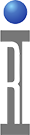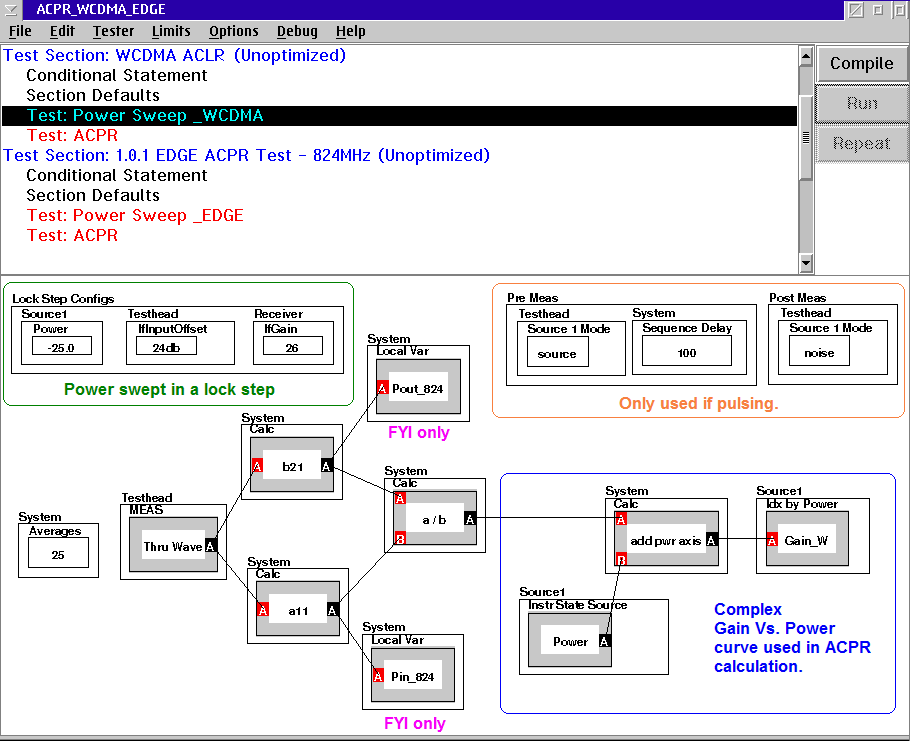ACPR is the Adjacent channel power ratio. A measurement of the amount of signal, or power, in the frequencies adjacent to the primary signal. ACPR is usually defined as the ratio of the average power in the adjacent frequency channel (or offset) to the average power in the transmitted frequency channel. It is a critical measurement for CDMA transmitters and their components. It describes the amount of distortion generated due to nonlinearities in RF components. The following example uses WCDMA, EDGE, and WiMAX signals to demonstrate how ACPR is measured using the model based approach. The waveform models used can be created by defining their parameters or by using a specific I/Q file imported into Guru.

1) Activate “Data Simulator”:

The Data Simulator is needed to provide the viewer with the modulation parameter buttons needed for the model based measurements. If your system already has the Data Simulator activated, then you will see an instrument called "DataSim" in your system configuration. If you do not see this in the list, then you will need to activate this virtual instrument.

To activate a Data Simulator on Cassini, click on the big 'System' button and then select 'Tester'. In the tester configuration panel click on 'Instrument' and select 'Add Inst…' from the drop down menu. Then choose the 'Data Simulator' from the list. Follow with a 'System'/ 'Check'. If you follow up by selecting 'Tester'/'Save', then the DataSim will be saved as part of the system configuration, and will automatically load when the system is activated.

2) Obtain the Complex Gain vs. Power Curve:

The main two parts of calculating the ACPR is to first do a power sweep and second do a calculation. The power sweep needs to be a 'Thru Wave' measurement. The "Thru Wave" calculation is a quick way to derive a complex value for b2/a1, which does not include any error correction. There is also no reference associated with the "Thru Wave" measurement, so the output is a relative phase rather than an absolute phase quantity. This fast, relative measurement is good for calculating AM/AM and AM/PM.

The complex gain curve produced from the "Thru Wave" measurement is then saved vs. input power. The example below shows some tips for pulsing or how to find the output power and input power, but these buttons can be removed if you are not planning on using them. The lockstep should be setup so that the output power vs. input power gives you a nice smooth curve from the linear region all the way into saturation. The curve needs to be at least long enough to account for the peak-to-average ratio (PAR). Note the "IFInputOffset" button which is used to account for the gain of the part and for measuring the b2 and a1 near the same power level. For more information on how to create a lockstep, look at the document "Lock Step Configs from CSV". Also, the numbers associated with the "b21" and "a11" stand for which port the signal is measured on followed by which port the signal is driven in on.There are two ways to implement the ACPR calculation. The first method shown below defines each specific modulation parameters (such as the modulation type, sample rate, number of symbols, etc.). Another way of implementing the ACPR calculation is by importing the specific waveform file. The two methods are described below:

a) Defining the Modulations:
• WCDMA
• The modulation type used for this example is HPSK (reverse uplink) with a sample rate of 30.72MHz(3.84Mcps x8), an ACP filter (which is a root raised cosine filter with α=0.22), 32 data points (or symbols), and 256 sample points.The “Adjust Power...” function adjusts the amplitude and phase by the nonlinearity in order to correct the average power for the modulated signal. The inputs are the modulated signal, the complex gain curve, and the desired output power. The following is the WCDMA IQ modulated waveform:The output is the ACPR, and the channel frequency range must be selected using the “Power from A to C” buttons. The two inputs to this button correspond to the upper and lower limits of the measurement bandwidth.

The ranges for WCDMA were based off of a bandwidth of 3.84MHz where the first adjacent channel was centered around 5MHz and the second around 10MHz.• EDGE

The modulation specifications used for EDGE are defined in the corresponding calculation panel: 8PSK , GMSK filter, 2.166MHz (270.833kbpsx8) sample rate, 32 symbols, and 256 sample points. For the example below: channel 1 is centered around +/- 400kHz and channel 2 centered around +/-600kHz. Since the bandwidth is so narrow and sensitive to level, the frequency range of the adjacent channels may need to be adjusted slightly.• WiMAX

The modulation specifications used for WiMAX are similar to the previous definitions; however, the Modulation Type gets switched from "Single Carrier" to "WiMAX". In this example the settings are: QPSK, 80MHz sample rate, 8192 symbols, 8192 sample points, and no filter. For the example below: channel 1 is centered around +/- 6MHz and channel 2 centered around +/- 10.5MHz, each with a 100kHz bandwidth .b) Using a Waveform File:

In order to use a specific waveform file, it first must be converted to a .csv, which can be done in Excel, Matlab, or a similar program. Then the .csv file needs to be imported into Guru. In order to do this the waveform file must first be placed on a drive that is accessible. The .csv file must be two columns of data in the form of I and Q and the amplitude of the waveform should be normalized from +1 to -1. To normalize the MAX/MIN to +/-1, divide all data by the largest (+ or -) value in the file.

To begin the import process, type into the main window "RiDataSim convertWaveform" and then highlight the text and right-mouse-button-click (RMBC) and select "Do It":This will then bring up a window asking to define the final waveform size. It can be as large as your like, however, the larger the file, the longer the tetsplan will take to compile and run. You will also be asked to define the start address, which pertains to the row from your original file that you want to start the waveform from. You must also define the number of input to output samples, or the number of every "x" samples that you want in your file. For example, if you select 4, that will choose every 4th sample in your file. Lastly, you will be prompted to find the .csv waveform file which you want to convert. Find the drive where you saved it and select "open". Next, re-title the waveform as you want to see it in Guru. This new waveform file will be saved as a RiWaveform file in Guru. To view the Guru waveform file, go to "Browse Guru" and scroll down to "Ri Waveform"; highlight it and right-click and select "edit" to view the details of the newly created file.

In order to use this waveform file in the calculation panel, change the 'Modulation Type' to "Guru File" and then select the file name from the 'Modulation File' button (as in the example below, the file name is "WiMAX"). Next, set the sample rate, and sample points; however, the number of symbols does not need to be defined when using the waveform from a file.https://roos.com/docs/JLUT-7SFURJ
ROOS INSTRUMENTS CONFIDENTIAL AND PROPRIETARY